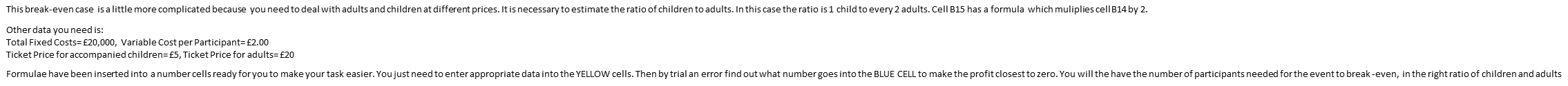# Hi guys please if you are given fixed cost of 20000 and price for child is 5 and for adult is 20,wh

Hi guys , please if you are given fixed cost of 20000 and price for child is 5 and for adult is 20,what will be the break-even, should two adults one child,variable cost is 2per

• Sales less variable costs equal contribution. Fixed costs divide by contribution will give you the breakeven number.
• Hi Krisso, This the full question
This break-even case is a little more complicated because you need to deal with adults and children at different prices. It is necessary to estimate the ratio of children to adults. In this case the ratio is 1 child to every 2 adults. Cell B15 has a formula which muliplies cell B14 by 2.

Other data you need is:
Total Fixed Costs= £20,000, Variable Cost per Participant= £2.00
Ticket Price for accompanied children= £5, Ticket Price for adults= £20

Formulae have been inserted into a number cells ready for you to make your task easier. You just need to enter appropriate data into the YELLOW cells. Then by trial an error find out what number goes into the BLUE CELL to make the profit closest to zero. You will the have the number of participants needed for the event to break -even, in the right ratio of children and adults• On that basis I would personally do the following

Fixed Costs / 3 x 2 Adults = £13333 (Rounded)
Fixed Costs / 3 (no need to multiply child) = £6667 (Rounded)

You can then do the adult and child calcs as follows

Adult Fixed Costs £13333 / 18 (Adult Contribution) = 740.7222 but Round up to the next whole number
Child Fixed Costs £6667 / 3 (Child Contribution) = 2222.33333 again round up to the next whole number

Hope this helps

• Hi Krisso, fixed cost is fixed, it does not change so in this case how do I go about it?
Thanks
• Unless I am reading the question incorrectly you are being requested to apportion the fixed costs to 2 adults and 1 child these your thus my calculation of fixed costs above is correct. I haven’t changed the fixed cost amount it still totals 20000. Fixed costs / contribution will give you break even figure for units and to break even you need to sell whole units so would have to round up for each calculation. From there you can then work out margin of safety in units, breakeven sales figures etc etc
• You don't split the fixed costs. One group contributes more than the other so doing a split fixed cost in the ratio of adults:children will not get you the break even value. Instead you need to work out the average contribution per attendee and apply that to the whole fixed cost.

Average contribution

=(18*2 +3)/3 =£13

Attendees needed

=20,000/13 = 1538.46 Rounds up 1539

Ratio

Children = 513

Check

1026 * 18 + 513 *3 = 18468 +1539 = 20007

You could get closer be removing only 1 child but you have to maintain the ratio so you'd also have to remove 2 adults.

1024 * 18 + 512 * 3 = 18432 +1536 = 19968

AAT Level 4, MAAT
ACCA in progress
F4- Passed Aug 2020
F5- Passed Dec 2020
F6- Passed Sep 2020
F7- Passed June 2021
F8 - Passed Sep 2021
F9 - Passed June 2021

SBL
SBR
ATX - Planned Dec 21
APM -